# Dimensional Analysis for Estimation of Convection Heat Transfer Coefficient - 2 - MCQs with Answers

## Dimensional Analysis for Estimation of Convection Heat Transfer Coefficient – 2 – MCQs with Answers

1. Experiment is carried out for the flow of fluid through a tube with various fluid velocities and convection heat transfer coefficients are measured for each velocity. How is the value of convection heat transfer coefficient varies with respect to velocity of the fluid?

a.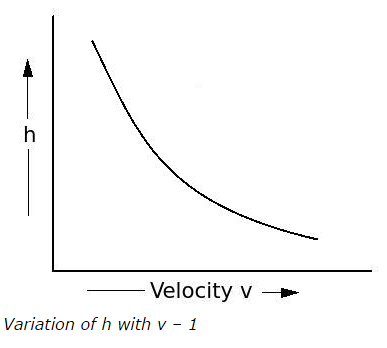b.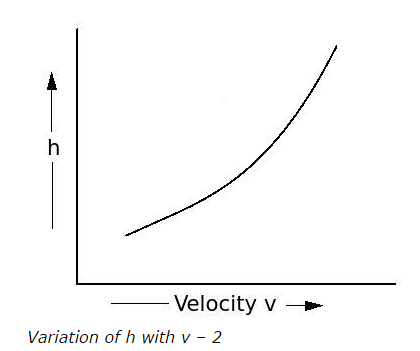c.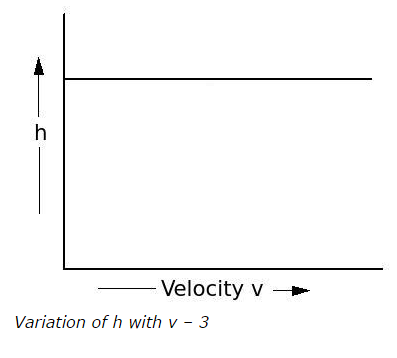d.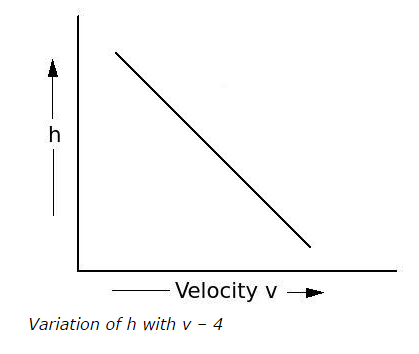2. How does the Nusselt number vary with variation of Reynolds number?

a.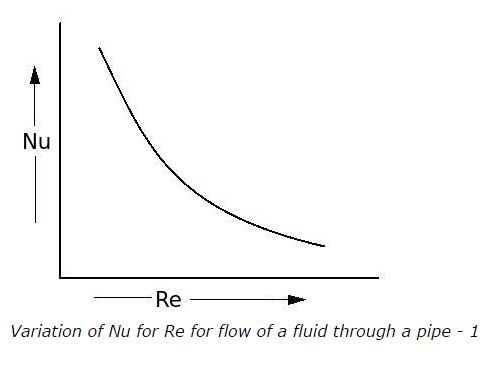b.c.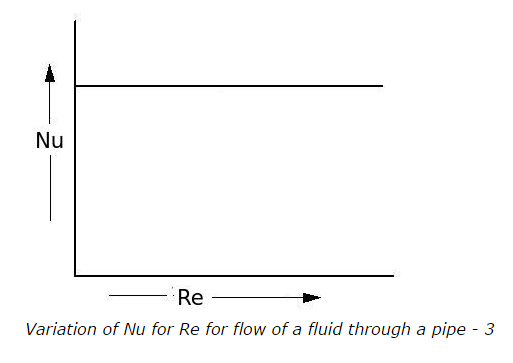d.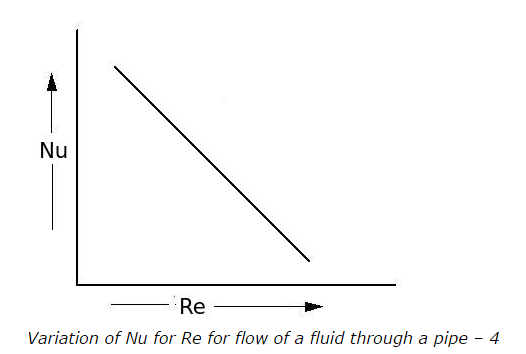3. The principle of similarity or model law states that

a. if the ratios of linear dimensions, forces, velocities, etc. of two systems are the same, then their behavior will be different as per the dimensions
b. if the ratios of linear dimensions, forces, velocities, etc. of two systems are the same, then their behavior will be similar
c. if the ratios of linear dimensions, forces, velocities, etc. of two systems are the different, then only their behavior will be similar
d. none of the above

ANSWER: b. if the ratios of linear dimensions, forces, velocities, etc. of two systems are the same, then their behavior will be similar

4. Prandtl number is the ratio of

a. thermal diffusivity (α) to absolute viscosity (μ)
b. absolute viscosity (μ) to thermal diffusivity (α)
c. kinematic viscosity (ν) to thermal diffusivity (α)
d. thermal diffusivity (α) to kinematic viscosity (ν)